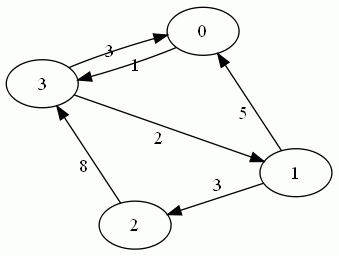## Monday, January 23, 2017

### Intermediate Algorithms: Directed Graphs

Today's problem is a relatively "simple" directed graph problem. In this problem we just want to find if there exists a route between two given nodes. Now, if you remember, a directed graph is one where the edges have *direction*, that is, nodes will not necessarily point back to the nodes that you traversed to get to them.An example of a directed graph

Now this problem is all about the edge cases. For example, we need to account for internal loops, as well as for running into dead ends. Now for the purposes of this problem, we're going to assume we're allowed to modify the nodes as we visit them. This is really a matter of convenience, if we could not alter the nodes, we would just have to modify our approach slightly.

At a high level, what we're going to do is build a BFS, utilizing a queue, and keep track of visited nodes by giving them a visited property. We could achieve that effect by keeping a list of visited nodes but it would affect our performance time by requiring us to iterate over it each time we visited a node.

To start, we'll begin at one of the nodes, and traverse the graph until we either find the node we're looking for, or run out of nodes to visit.

Lets look at what the code for this would look like, in javascript:

The algorithm will perform in O(n) time as it must theoretically traverse every node in a worst case scenario, but it has a memory complexity of O(1) as we're not storing anything other than state variables, this changes to O(n^2) and O(n) if we can't modify the nodes as we visit them.

If you wrote an algorithm and would like to test it, here's a super simple graph. 1 and 3 are connected, 3 and 1 are not.

## Sunday, January 15, 2017

### Intermediate Algorithms: Balanced Binary Beast

Algorithms continue to be a fun little pastime that is vital to people interviewing in the CS industry. We've covered some simple array algorithms, so its time for something a little more... interesting, my favorite data structure, trees. We're going to start with generating a tree. Not just any tree though, a balanced tree.

This exercise should take about 20 minutes.

So here we go: given a sorted array of numbers for example [1,3,5,8, 120], or [1,2,3,4,5,6], make a balanced binary tree. A balanced tree is one where the minimum depth and the maximum depth do not have a difference greater than one, or in other words where no "branches" are deeper than any other branches by one level. A binary tree is one in which each node has 0, 1 or 2 children. Unlike in this picture, our binary tree will be organized in such a way that the left child of each node will be greater than its parent, and the right child will be greater, making it useful for purposes of searching.

For the purposes of this exercise we'll be using javascript, as its more bare bones than ruby and syntactically closer to bare metal languages, you could easily however implement this in ruby as well. Of note is the fact that this algorithm cannot exceed O(n) time as we must iterate over the entire original array. An optimal solution then will have O(n) time.

Lets think through a solution: The first parameter to consider is our input, which is a sorted list.  By default we want our root to be the middle value of this sorted list, since this way we can just grab a value to right to be the first child, and a value to the left to be the second. Not just any value, because we want the children of the children to follow the same pattern as the root we want to grab the middle of the list.  Essentially we'll split the list in half with and then split the list in half again... does this smell like recursion? It does! So we'll make a node creation queue recursively, and then just go through it and make the nodes. Seems simple enough.

Now here's the code that makes the magic happen:

Not so bad is it? The bottom line, as long as you think through an algorithm, you can figure out a simple solution to the problem!